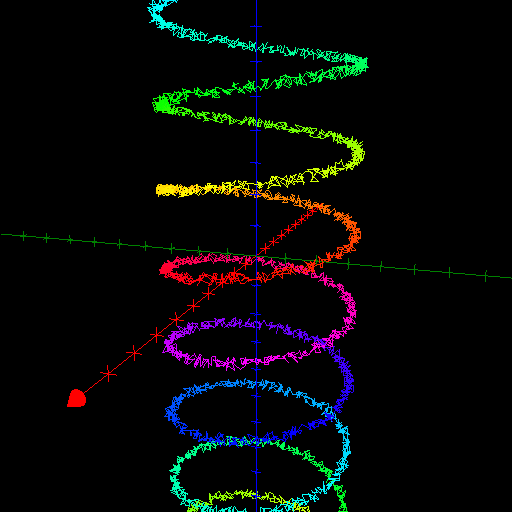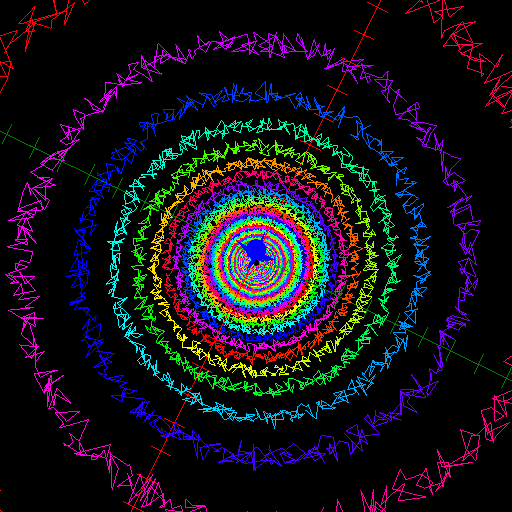$\newcommand{\sech}{\mathop{\rm sech}\nolimits} \newcommand{\csch}{\mathop{\rm csch}\nolimits} \newcommand{\R}{\mathbb{R}} \newcommand{\basis}{\underline{\mathbf{e}}}$
Current Page
Cylindrical helix

# Cylindrical helix

Object type: Space curve

### Definition

In $\mathbb{R}^3$, an elliptic cylindrical helix is an image $\mathbf{r} (\mathbb{R})$ where $$\mathbf{r}(t) = \underline{\mathbf{e}}\begin{pmatrix}a \cos{t} \\b \sin{t}\\ct\end{pmatrix}, \quad\quad\forall t\in\mathbb{R},$$ for some constants $a, b, c > 0$. If $a = b =: r$, we have a circular cylindrical helix of radius $r$. Notice that the elliptic (circular) cylindrical helix is a subset of the elliptic (circular) cylinder $$\left(\frac{x}{a}\right)^2 + \left( \frac{y}{b}\right)^2 = 1$$ which explains the name of the curve. Below a circular cylindrical helix with $r = 5$ and $c = 1/2$ is shown.### Arc Length

The length of a single 'turn' of an elliptic cylindrical helix, that is, the length of the curve $\mathbf{r}\left(\left]t_0, t_0 + 2\pi\right[\right)$ for some $t_0 \in \mathbb{R}$ is $$\int_{t_0}^{t_0 + 2\pi} {\sqrt{a^2 \sin^2 t + b^2 \cos^2 t + c^2} dt}.$$ In the case of a circular cylindrical helix, this reduces to $2 \pi \sqrt{r^2 + c^2}$.

### Curvature

The curvature function for the elliptic cylindrical helix (parameterised as above) is $$\kappa(t) = \frac{\sqrt{b^2 c^2 \sin^2 t + a^2 c^2 \cos^2 t + a^2 b^2}}{\left(a^2 \sin^2 t + b^2 \cos^2 t + c^2\right)^{3/2}}.$$ In the case of a circular cylindrical helix, this reduces to $$\kappa(t) = \frac{r}{r^2 + c^2}.$$

### Torsion

The torsion function for the elliptic cylindrical helix (parameterised as above) is $$\tau(t) = \frac{abc}{r^2 \left(r^2 + c^2\right)}.$$ In the case of a circular cylindrical helix, this reduces to $$\tau(t) = \frac{c}{r^2 + c^2}.$$

### Trivia

It is tempting to make a coloured and fuzzy helix.